# 图像特征提取（四）——FAST算法解析

https://blog.csdn.net/kezunhai/article/details/11290749

https://blog.csdn.net/hujingshuang/article/details/46898007

# 简介

在局部特征点检测快速发展的时候，人们对于特征的认识也越来越深入，近几年来许多学者提出了许许多多的特征检测算法及其改进算法，在众多的特征提取算法中，不乏涌现出佼佼者。

从最早期的Moravec，到Harris，再到SIFT、SUSAN、GLOH、SURF算法，可以说特征提取算法层出不穷。各种改进算法PCA-SIFT、ICA-SIFT、P-ASURF、R-ASURF、Radon-SIFT等也是搞得如火如荼，不亦乐乎。上面的算法如SIFT、SURF提取到的特征也是非常优秀（有较强的不变性），但是时间消耗依然很大，而在一个系统中，特征提取仅仅是一部分，还要进行诸如配准、提纯、融合等后续算法。这使得实时性不好，降系了统性能。

Edward Rosten和Tom Drummond两位大神经过研究，于2006年在《Machine learning for high-speed corner detection》中提出了一种FAST特征点，并在2010年稍作修改后发表了《Features From Accelerated Segment Test》，简称FAST。由于不涉及尺度，梯度，等复杂运算，Fast检测器速度非常快。它使用一定邻域内像元的灰度值与中心点比较大小去判断是否为一个角点。但它的缺点是不具有方向性,尺度不变性

# FAST详解

FAST：选择的特征点很接近角点
比较中间点与半径3.4的圆经过的邻域上的连续的12/9个点的灰度值，若有连续12或9个邻域像素值都大于或都小于中心像素点，则认为是候选特征点；用ID3信息增益进行决策树的训练；通过定义特征点响应函数进行角点的非极大值抑制；配合金字塔模型，可以对不同尺度的图像进行特征点检测，结果总和为最终结果；

## FAST特征的定义

FAST的提出者Rosten等将FAST角点定义为：若某像素与其周围邻域内足够多的像素点相差较大，则该像素可能是角点。

## FAST算法的步骤

FAST算法包含3个主要步骤：

（1）对固定半径圆上的像素进行分割测试，通过逻辑测试可以去处大量的非特征候选点；

（2）基于分类的角点特征检测，利用ID3 分类器根据16个特征判决候选点是否为角点特征，每个特征的状态为一1，0，1。

（3）利用非极大值抑制进行角点特征的验证。

1）固定半径圆上的像素进行分割测试（Segment Test）

在分割测试中，可以去除大量的非候选角点，这样就可以把可能的角点帅选出来。分割测试是通过对一固定半径的圆形模板的比较和计算进行的，在FAST角点检测算子中，一般是通过半径为3.4 pixel、外围16个像素的圆的作为模板，通过下图我们来具体分析下分割测试。

Fast角点提取步骤（以Fast-12-16为例）：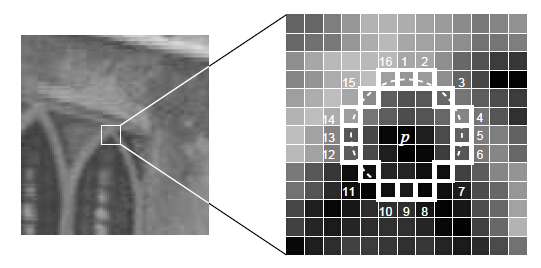1.以固定半径为圆的边上取16个像素点（图中白色框出的位置），与中心点像素值Ip做差。2.若边上存在连续的12（N>12,若为Fast-9,只需要N>9）个点满足  ( I(x)-I(p) )>threshold 或者 ( I(x)-I(p) ) < -threshold。(其中I(x)表示边上的像素值，I(p)为中心点像素值，threshold为设定的阈值。)则此点作为一个候选角点。为什么选择N =12点，因为通过测试，12点的角点检测性能最稳定、速度更快、效果也很好，当然有些文献指出9点的方式也很好。分割测试是怎么进行的呢？用下面的公式来说，便可一目了然。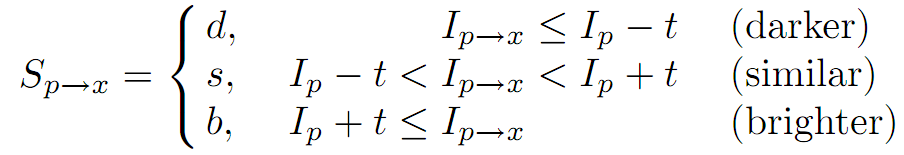上式中，t是一个阈值（默认取值为10，不同场景取值有差异），Ip表示的是中心像素的像素值，Ip->x表示的是圆形模板中的像素值上式的意思是：当中心像素的像素值Ip小于x处的像素值Ip->x+t时，则该像素属于darker，Sp->x=d，其他两种情况分别表示亮些和相似。这样一个块（圆形）区域就可以分成d、s和b三种类型。这时候只要统计圆形区域中d或b的次数，只要d或b出现的次数大于n（（当是12点分割测试角点检测时，n=12；当是9点时，则n=9），那么该点就被认为是候选角点。

在分割测试步骤中，为了加快速度，其实不需要对这些像素进行逐一的比较。简单来说：首先比较1、5、9、13处点的像素值（也即水平方向和垂直方向上的4个点）与中心像素值的大小，如果这四个点中的像素值有3个或3个以上大于Ip+t或小于Ip-t，那么则认为该处是一个候选角点，否则就不可能是角点。

2）ID3决策树算法来训练角点检测

通过上式中比较，可以将模板内的像素分成三部分d、s、b，分别记为：Pd，Ps，Pb。因此对于每个Sp->x都属于Pd，Ps，Pb中的一个。另外，令Kp为true，如果p为角点，否则为false。通过ID3算法来选择具有最大信息增益的像素来判断一个像素是否为角点。Kp的熵用下式来计算：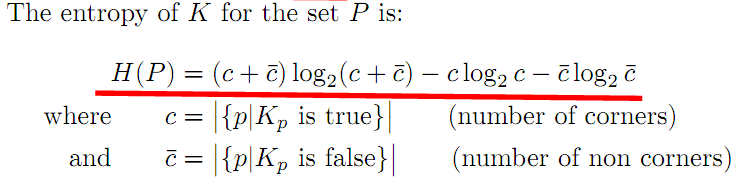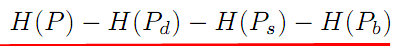3）非极大值抑制：排除不稳定角点

在上面的分割测试中，没有计算角点响应函数（Corner Response Function），非极大值抑制无法直接应用于提取的特征。因此，定义一个角点响应函数V，考虑到分割测试的特征以及计算速度的需要，角点响应函数的定义如下：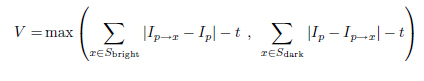总结：FAST角点检测算法是一种具有高计算效率(computational performance)、高可重复性(high repeatability)特征提取算子，在立体图像匹配、图像配准、目标识别、目标跟踪、场景重构等领域得到了广泛的应用，成为计算机视觉领域最流行的角点检测方法。但是，噪声对该算子的影响比较大，而且阈值t对算子的影响比较大。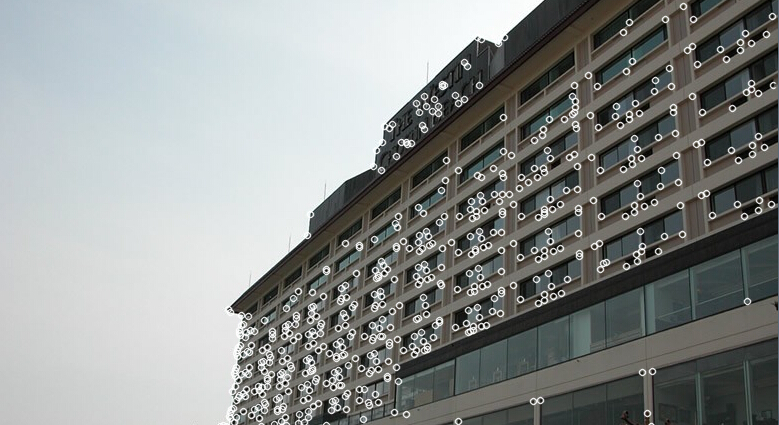OpenCV源码解析

[cpp] view plain copy

1. #include <stdio.h>
2. #include <iostream>
3. #include "cv.h"
4. #include "opencv2/highgui/highgui.hpp"
5. #include "opencv2/core/core.hpp"
6. #include "opencv2/features2d/features2d.hpp"
7. using namespace std;
8. using namespace cv;
9. int main( int argc, char** argv )
10. {
11.     Mat img_1 = imread( "F:\\Picture\\hotel.jpg");
12.     if( !img_1.data )
13.     {
14.         return -1; }
15.     FastFeatureDetector detector(50,true);  //第一个参数为阈值，第二个采用非最大值抑制
16.     std::vector<KeyPoint> keypoints_1;
17.     detector.detect( img_1, keypoints_1 );//调用FAST_t函数检测，见下面详细解析
18.     drawKeypoints(img_1,keypoints_1,img_1,Scalar::all(255));
19.     imshow("HOTEL",img_1);
20.     waitKey(0);
21.     return 0;
22. }

[cpp] view plain copy

1. void FAST_t(InputArray _img, std::vector<KeyPoint>& keypoints, int threshold, bool nonmax_suppression)
2. {
3.     Mat img = _img.getMat();
4.     const int K = patternSize/2, N = patternSize + K + 1;
5.
6.     int i, j, k, pixel;
7.     makeOffsets(pixel, (int)img.step, patternSize);
8.
9.     keypoints.clear();
10.
11.     threshold = std::min(std::max(threshold, 0), 255);//保证阈值在0-255之间。
12.
13.
14.     uchar threshold_tab;
15.     for( i = -255; i <= 255; i++ )
16.         threshold_tab[i+255] = (uchar)(i < -threshold ? 1 : i > threshold ? 2 : 0);  //分类成为darker、similar、brighter三种
17.
18.     AutoBuffer<uchar> _buf((img.cols+16)*3*(sizeof(int) + sizeof(uchar)) + 128);
19.     uchar* buf;
20.     buf = _buf; buf = buf + img.cols; buf = buf + img.cols;//保存对应角点强度值，否则为0
21.     int* cpbuf;
22.     cpbuf = (int*)alignPtr(buf + img.cols, sizeof(int)) + 1;//保存角点位置，+1为了存储这一行的角点总数
23.     cpbuf = cpbuf + img.cols + 1;
24.     cpbuf = cpbuf + img.cols + 1;
25.     memset(buf, 0, img.cols*3);
26.
27.     for(i = 3; i < img.rows-2; i++)
28.     {
29.         const uchar* ptr = img.ptr<uchar>(i) + 3;
30.         uchar* curr = buf[(i - 3)%3];
31.         int* cornerpos = cpbuf[(i - 3)%3];
32.         memset(curr, 0, img.cols);
33.         int ncorners = 0;
34.
35.         if( i < img.rows - 3 )
36.         {
37.             j = 3;
38.             /*采用9点分割测试，加快检测速度
39.               检测任意一个直径两端的像素点，若同时与中心点相似，必定不是角点
40.               因为至少要占一半的数量
41.             */
42.             for( ; j < img.cols - 3; j++, ptr++ )
43.             {
44.                 int v = ptr;
45.                 const uchar* tab = &threshold_tab - v + 255;
46.       int d = tab[ptr[pixel]] | tab[ptr[pixel]];//
47.                 if( d == 0 )      // 加快检测速度与两个点都与中心点灰度值相近，排除这个点
48.                     continue;
49.
50.                 d &= tab[ptr[pixel]] | tab[ptr[pixel]];//直径两端两个点都相近，则为0
51.                 d &= tab[ptr[pixel]] | tab[ptr[pixel]];//
52.                 d &= tab[ptr[pixel]] | tab[ptr[pixel]];//每隔45度选取一个点
53.
54.                 if( d == 0 )    //
55.                     continue;
56.
57.                 d &= tab[ptr[pixel]] | tab[ptr[pixel]];
58.                 d &= tab[ptr[pixel]] | tab[ptr[pixel]];
59.                 d &= tab[ptr[pixel]] | tab[ptr[pixel]];
60.                 d &= tab[ptr[pixel]] | tab[ptr[pixel]];
61.
62.                 if( d & 1 )   //     darker   中心值大，周围小的情况
63.                 {
64.                     int vt = v - threshold, count = 0;
65.
66.                     for( k = 0; k < N; k++ )   //且连续一半的像素点灰度差值( v-x > threshold )大于阈值
67.                     {
68.                         int x = ptr[pixel[k]];
69.                         if(x < vt)
70.                         {
71.                             if( ++count > K )
72.                             {
73.                              cornerpos[ncorners++] = j;
74.                              if(nonmax_suppression)//非最大值抑制
75.                         curr[j] = (uchar)cornerScore<patternSize>(ptr, pixel, threshold);//计算角点的强度响应值,最小的差值（绝对值）
76.                             break;
77.                             }
78.                         }
79.                         else
80.                             count = 0;
81.                     }
82.                 }
83.
84.                 if( d & 2 )//brighter 中心值小，周围值大的情况
85.                 {
86.                     int vt = v + threshold, count = 0;
87.
88.                     for( k = 0; k < N; k++ )   //连续一半的像素点灰度差值(  x-v < threshold )大于阈值
89.                     {
90.                         int x = ptr[pixel[k]];
91.                         if(x > vt)
92.                         {
93.                             if( ++count > K )
94.                             {
95.                             cornerpos[ncorners++] = j;
96.                              if(nonmax_suppression)
97.                         curr[j] = (uchar)cornerScore<patternSize>(ptr, pixel, threshold);//计算角点的强度响应值,最小的差值（绝对值）
98.                             break;
99.                             }
100.                         }
101.                         else
102.                             count = 0;
103.                     }
104.                 }
105.             }
106.         }
107.
108.         cornerpos[-1] = ncorners;//存储第i行上的角点总数量
109.
110.         if( i == 3 )
111.             continue;
112.        /*与邻域的8个角点响应值做比较，非角点的响应值为0*/
113.         const uchar* prev = buf[(i - 4 + 3)%3];  //相邻的两行
114.         const uchar* pprev = buf[(i - 5 + 3)%3];//
115.         cornerpos = cpbuf[(i - 4 + 3)%3];//存储角点的列位置
116.         ncorners = cornerpos[-1]; //存储第i行上的角点总数量
117.
118.         for( k = 0; k < ncorners; k++ )
119.         {
120.             j = cornerpos[k];
121.             int score = prev[j];
122.             if( !nonmax_suppression ||    //非极大值抑制,用角点强度值比较周围8个强度响应值
123.                (score > prev[j+1] && score > prev[j-1] &&
124.                 score > pprev[j-1] && score > pprev[j] && score > pprev[j+1] &&
125.                 score > curr[j-1] && score > curr[j] && score > curr[j+1]) )
126.             {
127.         keypoints.push_back(KeyPoint((float)j, (float)(i-1), 7.f, -1, (float)score));
128.             }
129.         }
130.     }
131. }

[cpp] view plain copy

1. int cornerScore<16>(const uchar* ptr, const int pixel[], int threshold)//角点强度计算
2. {
3.     const int K = 8, N = K*3 + 1;
4.     int k, v = ptr;
5.     short d[N];
6.     for( k = 0; k < N; k++ )    //计算与周围16个像素点的差值,保存在d[k]中
7.         d[k] = (short)(v - ptr[pixel[k]]);
8.     int a0 = threshold;
9.     for( k = 0; k < 16; k += 2 )  //周围像素小于中心点像素
10.     {
11.         int a = std::min((int)d[k+1], (int)d[k+2]);
12.         a = std::min(a, (int)d[k+3]);
13.         if( a <= a0 )
14.             continue;
15.         a = std::min(a, (int)d[k+4]);
16.         a = std::min(a, (int)d[k+5]);
17.         a = std::min(a, (int)d[k+6]);
18.         a = std::min(a, (int)d[k+7]);
19.         a = std::min(a, (int)d[k+8]);
20.         a0 = std::max(a0, std::min(a, (int)d[k]));
21.         a0 = std::max(a0, std::min(a, (int)d[k+9]));
22.     }
23.
24.     int b0 = -a0;
25.     for( k = 0; k < 16; k += 2 )//周围像素点大于中心像素点
26.     {
27.         int b = std::max((int)d[k+1], (int)d[k+2]);
28.         b = std::max(b, (int)d[k+3]);
29.         b = std::max(b, (int)d[k+4]);
30.         b = std::max(b, (int)d[k+5]);
31.         if( b >= b0 )
32.             continue;
33.         b = std::max(b, (int)d[k+6]);
34.         b = std::max(b, (int)d[k+7]);
35.         b = std::max(b, (int)d[k+8]);
36.
37.         b0 = std::min(b0, std::max(b, (int)d[k]));
38.         b0 = std::min(b0, std::max(b, (int)d[k+9]));
39.     }
40.
41.     threshold = -b0-1;
42.
43.     return threshold;
44. }

# 实验

## opencv代码


1. #include <opencv2/core/core.hpp>

2. #include <opencv2/features2d/features2d.hpp>

3. #include <opencv2/highgui/highgui.hpp>

4. #include <cv.h>

5. #include <vector>

6.
7. using namespace cv;

8. using namespace std;

9.
10. int main()

11. {

12. Mat frame=imread("lena.jpg", 1);

13. double t = getTickCount();//当前滴答数

14. std::vector<KeyPoint> keyPoints;

15. FastFeatureDetector fast(50); // 检测的阈值为50

16.
17. fast.detect(frame, keyPoints);

18. drawKeypoints(frame, keyPoints, frame, Scalar(0,0,255), DrawMatchesFlags::DRAW_OVER_OUTIMG);

19.
20. t = ((double)getTickCount() - t)/getTickFrequency();

21. cout<<"算法用时："<<t<<"秒"<<endl;

22.
23. imshow("FAST特征点", frame);

24. cvWaitKey(0);

25.
26. return 0;

27. }## MATLAB代码


1. clear all;

2. close all;

3. %%

4. pic=imread('lena.jpg');

5. img=pic;

6. [M N D]=size(pic);

7. if D==3

8. pic=rgb2gray(pic);

9. end

10. %%

11. mask=[0 0 1 1 1 0 0;...

12. 0 1 0 0 0 1 0;...

13. 1 0 0 0 0 0 1;...

14. 1 0 0 0 0 0 1;...

15. 1 0 0 0 0 0 1;...

16. 0 1 0 0 0 1 0;...

17. 0 0 1 1 1 0 0];

18. mask=uint8(mask);

19. threshold=50;

20. figure;imshow(img);title('FAST角点检测');hold on;

21. tic;

22. for i=4:M-3

23. for j=4:N-3%若I1、I9与中心I0的差均小于阈值，则不是候选点

24. delta1=abs(pic(i-3,j)-pic(i,j))>threshold;

25. delta9=abs(pic(i+3,j)-pic(i,j))>threshold;

26. delta5=abs(pic(i,j+3)-pic(i,j))>threshold;

27. delta13=abs(pic(i,j-3)-pic(i,j))>threshold;

28. if sum([delta1 delta9 delta5 delta13])>=3

29. block=pic(i-3:i+3,j-3:j+3);

30. block=block.*mask;%提取圆周16个点

31. pos=find(block);

32. block1=abs(block(pos)-pic(i,j))/threshold;

33. block2=floor(block1);

34. res=find(block2);

35. if size(res,1)>=12

36. plot(j,i,'ro');

37. end

38. end

39. end

40. end

41. toc;

42. %%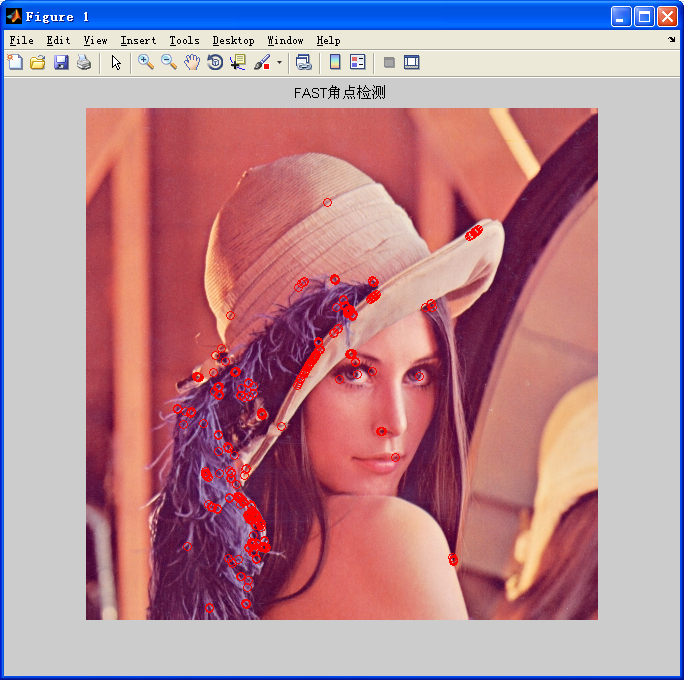# 参考文章

Edward Rosten et.:Machine Learning for High-Speed Corner Detection

http://blog.csdn.Net/kezunhai/article/details/11290749

http://www.edwardrosten.com/work/fast.html

1、FAST Corner Detection -- Edward Rosten：http://www.edwardrosten.com/work/fast.html

2、A Brief History of FAST corner detector：http://blog.csdn.net/anshan1984/article/details/8867653

3、AGAST Corner Detector: faster than FAST and even FAST-ER：http://www6.in.tum.de/Main/ResearchAgast

1、Machine learning for high-speed corner detection[J],2006.

2、Features From Accelerated Segment Test[J],2010.

3、基于自适应阈值的FAST特征点提取算法[J],2013

12-1905-256824
01-312万+
07-154万+
03-226232
11-201万+
05-105999
11-24
07-144680
11-18
12-12
05-03
04-211万+
02-144678
10-041万+
11-15382
©️2020 CSDN 皮肤主题: 大白 设计师:CSDN官方博客